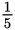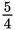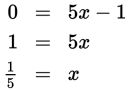# SAT Math Multiple Choice Question 791: Answer and Explanation

### Test Information

Question: 791

11. If the graph of the equation y = 5x + 3 is shifted down 4 units, what is the x-intercept of the new line?

• A. –1
• B.• C. 1
• D.Explanation:

B

Difficulty: Medium

Category: Heart of Algebra / Linear Equations

Strategic Advice: You aren't given a graph to shift, so either draw a quick sketch of the given line or mentally picture what happens when the line is shifted. Don't forget-when a linear equation is written in the form y = mx + b, m is the slope of the line and b is the y-intercept (not the x-intercept).

Getting to the Answer: The graph of the given line has a slope of 5 and a y-intercept of 3. Shifting the line down 4 units doesn't change the slope, but the y-intercept moves down 4, so the new y-intercept is 3 - 4 = -1. Wait, though-choice A is not the correct answer: The question asks for the x-intercept of the new line, not the y-intercept. The equation of the new line is y = 5x - 1. Set y = 0 and solve for x:This means (B) is correct.